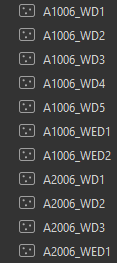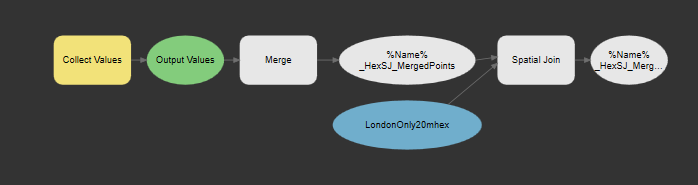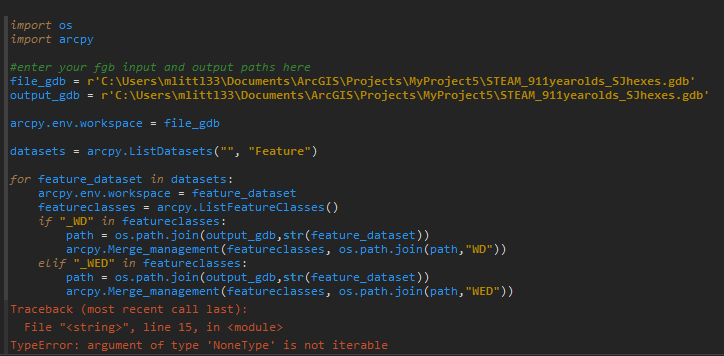# Loop through feature datasets in a gdb

5396
11
02-22-2020 01:27 PMNew Contributor II

Hello,

Im using modelBuilder to attempt to create polygon datasets for each feature dataset in a geodatabase. Each feature dataset has a set of point feature classes, some for Weekdays (*WD*) and some for Weekends (*WED*):I want to merge the point feature classes into one (based on wildcard for *WD* or *WED*), then perform a spatial join with an existing polygon feature. This is the model I have constructed so far:Thing is, whatever I attach to Collect Values, be it a submodel that Iterates Datasets, then feeds that into Iterate Feature Classes, there's no resulting spatially joined polygon feature, or the resulting polygon feature is including everything in the gdb.

What is required to merge just feature classes inside a feature dataset, then loop through a whole geodatabase full of feature datasets?

Tags (4)
1 Solution

Accepted SolutionsbyMVP Frequent Contributor
``````import os
import arcpy

#enter your fgb input and output paths here
file_gdb = r'your gdb to loop thru'
output_gdb = r'output gdb'

arcpy.env.workspace = file_gdb

datasets = arcpy.ListDatasets("", "Feature")

fd_path_list = [ ]
fd_name_dict = { }
for feature_dataset in datasets:
fd_path = os.path.join(file_gdb, feature_dataset)
fd_path_list.append(fd_path)
fd_name_dict[fd_path] = str(feature_dataset)

print(fd_name_dict)
for fd in fd_path_list:
fc_path_list_WD = [ ]
fc_path_list_WED = [ ]
arcpy.env.workspace = fd
featureclasses = arcpy.ListFeatureClasses()

for fc in featureclasses:

if "_WD" in fc:
fc_path = os.path.join(fd, fc)
fc_path_list_WD.append(fc_path)

if "_WED" in fc:
fc_path = os.path.join(fd, fc)
fc_path_list_WED.append(fc_path)

wd_path = os.path.join(output_gdb, fd_name_dict[fd])
print(fd_name_dict[fd])
wd_path = wd_path + "_WD"
print(wd_path)

if len(fc_path_list_WD) != 0:
try:
arcpy.Merge_management(fc_path_list_WD, wd_path)
except:
continue

wed_path = os.path.join(output_gdb, fd_name_dict[fd])
wed_path = wed_path + "_WED"
print(wed_path)

if len(fc_path_list_WED) != 0:
try:
arcpy.Merge_management(fc_path_list_WED, wed_path)
except:
continue

del fc_path_list_WD
del fc_path_list_WED

print("done")
‍‍‍‍‍‍‍‍‍‍‍‍‍‍‍‍‍‍‍‍‍‍‍‍‍‍‍‍‍‍‍‍‍‍‍‍‍‍‍‍‍‍‍‍‍‍‍‍‍‍‍‍‍‍‍‍‍‍‍‍‍‍‍‍‍‍‍‍‍‍‍‍‍‍‍‍‍‍‍‍‍‍‍‍‍‍‍‍‍‍‍‍‍‍‍‍‍‍‍‍‍‍‍‍‍‍‍‍‍‍‍‍‍‍‍‍‍‍‍‍‍‍‍‍‍‍‍‍‍‍‍‍‍‍‍‍‍‍‍‍‍‍‍‍‍‍‍‍‍‍‍‍‍‍‍‍‍‍‍‍‍‍‍‍‍‍‍‍‍‍‍‍‍‍‍‍‍‍‍‍‍‍‍‍‍‍‍‍‍‍‍‍‍‍‍‍‍‍‍‍‍‍‍‍‍‍‍‍‍‍‍‍‍‍‍‍‍‍‍‍‍‍‍‍‍‍‍‍‍‍``````
11 RepliesbyMVP Frequent Contributor

If you want those as a script it should be pretty easy and I'm happy to help, though I understand that might not be the point. I'm happy to admit my knowledge of model builder is limited!New Contributor II

Running scripts through the Python console is also acceptable.byMVP Frequent Contributor

The only thing is the spatial join. What are the shapefiles and how are they structured? I'll need to define this in the codeNew Contributor II

The point feature classes in this image are in their own feature datasets (e.g. A1006_subject feature dataset) in one geodatabase:The polygon target feature of the spatial join is located in another geodatabase.byMVP Frequent Contributor

Yes but I need to know what the target shapefiles look likeNew Contributor II

In all honesty, if the script can create merged point feature classes, the spatial joining portion I can easily run after, in a modelbuilder model.byMVP Frequent Contributor
``````import os
import arcpy

#enter your fgb input and output paths here
file_gdb = r'your gdb to loop thru'
output_gdb = r'output gdb'

arcpy.env.workspace = file_gdb

datasets = arcpy.ListDatasets("", "Feature")

fd_path_list = [ ]
fd_name_dict = { }
for feature_dataset in datasets:
fd_path = os.path.join(file_gdb, feature_dataset)
fd_path_list.append(fd_path)
fd_name_dict[fd_path] = str(feature_dataset)

print(fd_name_dict)
for fd in fd_path_list:
fc_path_list_WD = [ ]
fc_path_list_WED = [ ]
arcpy.env.workspace = fd
featureclasses = arcpy.ListFeatureClasses()

for fc in featureclasses:

if "_WD" in fc:
fc_path = os.path.join(fd, fc)
fc_path_list_WD.append(fc_path)

if "_WED" in fc:
fc_path = os.path.join(fd, fc)
fc_path_list_WED.append(fc_path)

wd_path = os.path.join(output_gdb, fd_name_dict[fd])
print(fd_name_dict[fd])
wd_path = wd_path + "_WD"
print(wd_path)

if len(fc_path_list_WD) != 0:
try:
arcpy.Merge_management(fc_path_list_WD, wd_path)
except:
continue

wed_path = os.path.join(output_gdb, fd_name_dict[fd])
wed_path = wed_path + "_WED"
print(wed_path)

if len(fc_path_list_WED) != 0:
try:
arcpy.Merge_management(fc_path_list_WED, wed_path)
except:
continue

del fc_path_list_WD
del fc_path_list_WED

print("done")
‍‍‍‍‍‍‍‍‍‍‍‍‍‍‍‍‍‍‍‍‍‍‍‍‍‍‍‍‍‍‍‍‍‍‍‍‍‍‍‍‍‍‍‍‍‍‍‍‍‍‍‍‍‍‍‍‍‍‍‍‍‍‍‍‍‍‍‍‍‍‍‍‍‍‍‍‍‍‍‍‍‍‍‍‍‍‍‍‍‍‍‍‍‍‍‍‍‍‍‍‍‍‍‍‍‍‍‍‍‍‍‍‍‍‍‍‍‍‍‍‍‍‍‍‍‍‍‍‍‍‍‍‍‍‍‍‍‍‍‍‍‍‍‍‍‍‍‍‍‍‍‍‍‍‍‍‍‍‍‍‍‍‍‍‍‍‍‍‍‍‍‍‍‍‍‍‍‍‍‍‍‍‍‍‍‍‍‍‍‍‍‍‍‍‍‍‍‍‍‍‍‍‍‍‍‍‍‍‍‍‍‍‍‍‍‍‍‍‍‍‍‍‍‍‍‍‍‍‍‍``````New Contributor II

Put that into the console, but it gave an error:I think the for loop doesn't retain information on the feature dataset location.byMVP Frequent Contributor

yes, thought the list() was retuning objects..

I've edited the code in my original reply which should work.